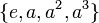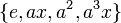# Fusion systems for dihedral group:D8

## Contents

View fusion systems for particular groups | View other specific information about dihedral group:D8

This article discusses the possible fusion systems for the dihedral group of order eight.$G = \langle a,x \mid a^4 = x^2 = e, xax^{-1} = a^{-1} \rangle$.

There are, up to isomorphism, two possible fusion systems on$G$.

## The inner fusion system: the fusion system obtained from inner automorphisms

This is the fusion system where all morphisms are obtained as the restriction of inner automorphisms of$G$. The isomorphisms are as follows.

Equivalence class under isomorphisms Subgroups involved Order Index Total number of subgroups Are all group automorphisms of each subgroup included? Size of automorphism group from the fusion system Total number of isomorphisms (including automorphisms and others) = (number of automorphisms)$\times$ (number of subgroups)${}^2$
trivial subgroup 1 8 1 Yes 1 1$\{ e, a^2 \}$ center of dihedral group:D8 2 4 1 Yes 1 1$\{ e, x \}, \{ e, a^2x \}$ non-normal subgroups of dihedral group:D8 (some of them) 2 4 2 Yes 1 4$\{ e, ax \}, \{ e, a^3x \}$ non-normal subgroups of dihedral group:D8 (some of them) 2 4 2 Yes 1 4$\{ e, a, a^2, a^3 \}$ cyclic maximal subgroup of dihedral group:D8 4 2 1 Yes 2 2$\{ e, x, a^2, a^2x \}$ Klein four-subgroups of dihedral group:D8 (one of them) 4 2 1 No 2 2$\{ e, ax, a^2, a^3x \}$ Klein four-subgroups of dihedral group:D8 (one of them) 4 2 1 No 2 2
whole group 8 1 1 No 4 4

## The other fusion system

This is unique up to automorphisms of the group. There are in fact two versions of this viewed strictly, which are interchanged under the outer automorphism sending$x$ to$ax$ and fixing$a$.

This fusion system is realized, for instance, in the symmetric group of degree four.

Equivalence class under isomorphisms Subgroups involved Order Index Total number of subgroups Are all group automorphisms of each subgroup included? Size of automorphism group from the fusion system Total number of isomorphisms (including automorphisms and others) = (number of automorphisms)$\times$ (number of subgroups)${}^2$
trivial subgroup 1 8 1 Yes 1 1$\{ e, a^2 \}, \{ e, x \}, \{ e, a^2x \}$ center of dihedral group:D8, non-normal subgroups of dihedral group:D8 (some of them) 2 4 3 Yes 1 9$\{ e, ax \}, \{ e, a^3x \}$ non-normal subgroups of dihedral group:D8 (some of them) 2 4 2 Yes 1 4$\{ e, a, a^2, a^3 \}$ cyclic maximal subgroup of dihedral group:D8 4 2 1 Yes 2 2$\{ e, x, a^2, a^2x \}$ Klein four-subgroups of dihedral group:D8 (one of them) 4 2 1 Yes 6 6$\{ e, ax, a^2, a^3x \}$ Klein four-subgroups of dihedral group:D8 (one of them) 4 2 1 No 2 2
whole group 8 1 1 No 4 4